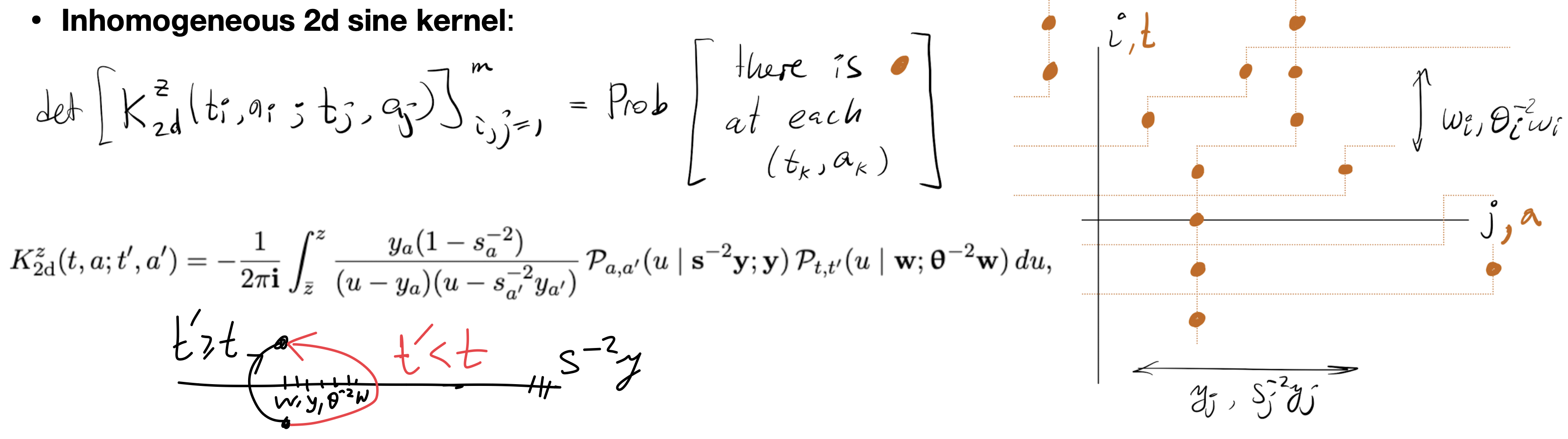# Schur rational functions, vertex models, and random tilings

Discusses symmetric functions and stochastic models based on the free fermionic six vertex model. Follows joint work with Aggarwal, Borodin, and Wheeler.

The work deals with symmetric rational functions and probabilistic models based on the fully inhomogeneous six vertex (ice type) model satisfying the free fermion condition. Two families of symmetric rational functions $F_\lambda,G_\lambda$ are defined as certain partition functions of the six vertex model, with variables corresponding to row rapidities, and the labeling signatures $\lambda=(\lambda_1\ge \ldots\ge \lambda_N)\in \mathbb{Z}^N$ encoding boundary conditions. These symmetric functions generalize Schur symmetric polynomials, as well as some of their variations, such as factorial and supersymmetric Schur polynomials. Cauchy type summation identities for $F_\lambda,G_\lambda$ and their skew counterparts follow from the Yang–Baxter equation. Using algebraic Bethe Ansatz, we obtain a double alternant type formula for $F_\lambda$ and a Sergeev–Pragacz type formula for $G_\lambda$.

In the spirit of the theory of Schur processes, we define probability measures on sequences of signatures with probability weights proportional to products of our symmetric functions. We show that these measures can be viewed as determinantal point processes, and we express their correlation kernels in a double contour integral form. We present two proofs: The first is a direct computation of Eynard–Mehta type, and the second uses non-standard, inhomogeneous versions of fermionic operators in a Fock space coming from the algebraic Bethe Ansatz for the six vertex model.

We also interpret our determinantal processes as random domino tilings of a half-strip with inhomogeneous domino weights. In the bulk, we show that the lattice asymptotic behavior of such domino tilings is described by a new determinantal point process on $\mathbb{Z}^{2}$, which can be viewed as an doubly-inhomogeneous generalization of the extended discrete sine process.

PDF (8.9 MB)Inhomogeneous two-dimensional discrete sine kernel